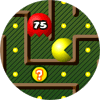Custom Search

# Find Our Cool Math Games to Play by Category:

### 4.NBT.B.5

Multiply a whole number of up to four digits by a one-digit whole number, and multiply two two-digit numbers, using strategies based on place value and the properties of operations. Illustrate and explain the calculation by using equations, rectangular arrays, and/or area models.CCSS: 4.NBT.B.5
Play Pac Man with a mathematical twist. Eat the power pill to reveal the equation and then eat the ghost that solves it.